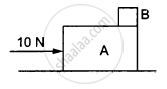# A Small Block B is Placed on Another Block a of Mass 5 Kg and Length 20 Cm. Initially, the Block B is Near the Right End of Block a (Figure 5−E3). a Constant Horizontal Force of 10 N is Applied - Physics

Sum

A small block B is placed on another block A of mass 5 kg and length 20 cm. Initially, the block B is near the right end of block A (In the following Figure). A constant horizontal force of 10 N is applied to the block A. All the surfaces are assumed frictionless. Find the time that elapses before block B separates from A.#### Solution

Mass of block A, m = 5 kg
F = ma = 10 N
$\Rightarrow a = \frac{10}{5} = 2 m/ s^2$
As there is no friction between A and B, when block A moves, block B remains at rest in its position.
Initial velocity of A, u = 0
Distance covered by A to separate out,
s = 0.2 m
Using $s = ut + \frac{1}{2}a t^2$
$0 . 2 = 0 + \frac{1}{2} \times 2 t^2$
⇒ t2 = 0.2
⇒ t = 0.44 s ≈ 0.45 s

Concept: Newton’s Second Law of Motion
Is there an error in this question or solution?

#### APPEARS IN

HC Verma Class 11, Class 12 Concepts of Physics Vol. 1
Chapter 5 Newton's Laws of Motion
Q 11 | Page 79